Bakery and flour

The bakery tray for flour was filled to 3/4 volume. After removing 875 kg of flour, it was filled to only 2/5 of the volume. How many tons of flour is in the full tray?

Result

x =  2500 t

Solution:

3/4 * x - 875 = 2/5 *x

7x = 17500

x = 2500

Calculated by our simple equation calculator.

Leave us a comment of this math problem and its solution (i.e. if it is still somewhat unclear...):Be the first to comment!To solve this verbal math problem are needed these knowledge from mathematics:

Do you have a linear equation or system of equations and looking for its solution? Or do you have quadratic equation?

Next similar math problems:

1. Bakery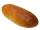How heavy must prepare bread at a bakery if lose during baking 16% of water and after baking must have 2 kg? (Calculate to the nearest gram)
2. CandyPeter had a sachet of candy. He wanted to share with his friends. If he gave them 30 candies, he would have 62 candies. If he gave them 40 candies, he would miss 8 candies. How many friends did Peter have?
3. Calcium and citality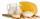The daily dose of calcium obtained using 0.75 liters of milk and 60 grams of cheese. A/ At the morning I drank half a liter of milk. How much cheese I must eatto eat a daily dose of calcium? b / I ate 50 grams of cheese, how much milk I must drink? c /.
4. CandyThe price for 1kg of more expensive candy is 125 CZK. The price for 1kg of cheaper candy is 100 CZK. We make two different blends of candy. And now. The second blend contains 2kg of more expensive candy and several kg of cheaper candy. The price per 1 kg o
5. Mushrooms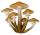For five days, we have collected 410 mushrooms. Interestingly every day we have collected 10 mushrooms more than the preceding day. How many mushrooms we have collected during 4th day?
6. Apples 2How many apples were originally on the tree, if the first day fell one third, the second day quarter of the rest and on tree remained 45 apples?
7. Tickets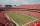On the football tournament ticket cost 45 Kc for standing and 120kč for sitting. Sitting spectators was 1/3 more than standing. The organizers collected a total 12 300 Kc. How many seated and standing seats (spectators)?
8. Three granaries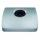Three granaries hold 7,800 metric cent (q) of grain. In the first, 70 tonnes of grain was more than the second and in the third it was 120 tonnes less than in the second. How many grain were in each granary?
9. Roses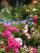On the large rosary was a third white, half red, yellow quarter and six pink. How many roses was in the rosary?
10. Sales storesThe first sales store passed the 1/3 and the second 2/5 of the total amount of goods.In third store passed the 2/3 rest of the goods. The remaining 40 kg of goods put into fourth store. How many kilograms of goods pass to a third store?
11. Unknown numberIdentify unknown number which 1/5 is 40 greater than one tenth of that number.
12. EquationSolve the equation: 1/2-2/8 = 1/10; Write the result as a decimal number.
13. Simple equationSolve for x: 3(x + 2) = x - 18
14. Fifth of the numberThe fifth of the number is by 24 less than that number. What is the number?
15. Heating plant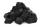Workers dump imported coke at heating plant. On the first day used half of the amount, the second day three quarters the rest and on the third day left 120 tons. How much coke they dump at heating plant?
16. Father and sonFather and son weigh together 108kg. The father weighs 2.6 times more than his son. How much does weight my father and son count?Number 118 divide into two addends, so first addend is 69 greater than 75% of the second addend.